Type Here to Get Search Results !

# PHYSICS FORM FOUR TOPIC 2: ELECTROMAGNETISM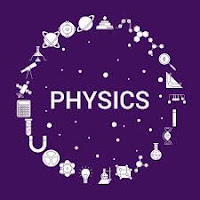ELECTROMAGNETISM
How Electric Current Produce a Magnetic Field
Explain how electric current produces a magnetic field
Electromagnetism is the effect produced by the interaction of an electric current with a magnetic field. The interaction can result in a force causing the conductor carrying the current.
If, on the other hand, a force is applied to a conductor (with no current) in a magnetic field the resulting movement can result in a current being noticed in the conductor.
When the switch is closed an electric current flows through the conductor. The electric current generates magnetic field around the conductor. This will cause a deflection on the compass needle. The magnetic field around a current-carrying conductor can be shown by means of magnetic field lines.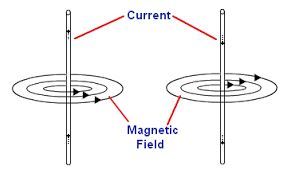The Pattern of the Magnetic Field Lines around a Straight Conductor
Identify the pattern of the magnetic field lines around a straight conductor
The magnetic field pattern is usually given in a plan view. In the plan view, the conductor is represented by a circle. A dot in circle shows that the current is coming out of the plane. A cross the circle shows that the current is moving into the plane.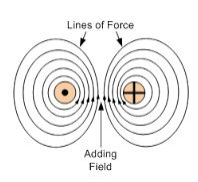The strength of the magnetic field on the magnitude of the electric current. The higher the current, the stronger the magnetic field, and therefore the greater the deflection. The strength of the magnetic field decreases as you move further from the conductor. There will be less deflection as the compass is drawn from the current-carrying conductor.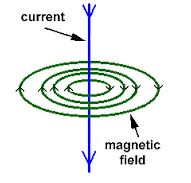The Direction of Magnetic Field around a Current-Carrying Conductor
Determine the direction of magnetic field around a current-carrying conductor
The direction of the field is determined by applying two rules, these are:
1. Right-hand Grip Rule
2. Maxwell’s cork screw rule
Right-hand Grip Rule
The Right-hand Grip Rule can be applied to a straight conductor or a solenoid-carrying an electric current. For a straight conductor, the Right-hand Grip Rule can be stated as:
“Imagine the wire carrying the current is gripped by the right hand with the thumb pointing in the direction of the conventional current (from positive to negative), the fingers will curl around the wire pointing in the direction of the magnetic field.”For a solenoid, the Right-hand Grip Rule states that:
“When you wrap your right hand around a solenoid with your fingers pointing in the direction of convectional current, your thumb point in the direction of the magnetic North pole.”
A solenoid is a long coil containing a large number of close turns of illustrated copper wire.
Maxwell’s –Right –hand screw rule states that:
“If a right-hand screw advances in the direction of the current, then the direction of rotation of the screw represents the direction of the magnetic field due to the current.”The Presence and Direction of a Force on a Current carrying Conductor in a Magnetic Field
Determine the presence and direction of a force on a current carrying Conductor in a magnetic field
The direction of the force on a current-carrying conductor in a magnetic field can be determined using Fleming’s Left –Hand Rule.
Fleming’s Left –Hand Rule states that:
“If you hold the index finger, the middle finger and the thumb of your left hand mutually perpendicular to each other so that the index finger points in the direction of the magnetic field and the middle finger points in the direction of current in the conductor, then the thumb will point in the direction of the force acting on the conductor.”The Direction of Force due to two Current carrying Conductors when the Current Flowing in the Same or Opposite Direction
Determine the direction of force due to two current '82air-carrying conductors when the current flowing in the same or opposite direction
If two current-carrying conductors are placed side by side close to one another, the currents in the conductors will interact with the magnetic fields produced by the two conductors. A force may result depending on the direction of the two currents.
When the currents are flowing in opposite directions, the conductors repel one another. When the currents are flowing in the same direction, the conductors attract one another, the conductors attract each other.
When the currents flow in the same direction, the magnetic field between the conductors cancel out, thus reducing the net field. However, on the outside, the magnetic fields add up, thus increasing the net field. Therefore, the magnetic field is weaker between the conductors that on the outside. The resultant force pushes the conductor towards each other.
When the currents are in the opposite directions, the fields between the conductors add up, while they cancel out on the outside. The field between them is stronger than on the outside. The resultant force is toward the outside of each conductor, hence repulsion.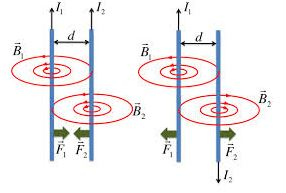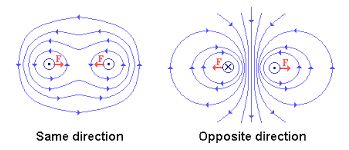The Concept of Electromagnetic Induction
Explain the concept of electroMagnetic induction
Electromagnetic Induction is the production of e. m. f whenever there is a change in the magnetic flux linking a conductor”. The e. m. f produced is called induced e. m. f and the resulting current induced current.
The Laws of ElectroMagnetic Induction
State the laws of electroMagnetic induction
Lenz’s Law
This explains the direction of the induced e. m. f and it states that: “The direction of the induced e. m. f is such that the resulting induced current flows in such a direction that it opposes the change that causes it.”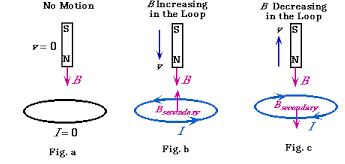It relates the magnitude of induced e. m. f and the rate of change of the magnetic flux linking the conductor. The magnitude of the induced e. m. f depends on:
• the strength of the magnetic field.
• the rate of change of the magnetic flux(speed of motion)
• the area of the conductor that is in the magnetic field.
Faraday’s law states that: “The e. m. f induced in the conductor in a magnetic field is proportional to the rate of change of magnetic flux linking the conductor.”
The Concepts of Self and Mutual Induction
Explain the concepts of self and mutual induction
When the current flowing through a conductor varies it creates a varying magnetic field that cuts across the conductor itself.
This results to self-induced e. m .f in the conductor that is opposite in direction to the original e. m. f. This voltage, usually referred to as back e. m .f, tends to limit or reverse the original current.
If the original current is increasing, then the induced current subtracts from it and then measured current is smaller than it would be if no self-induced magnetic field was produced in the conductor. If original current is decreasing, then the original current adds to it and the measured current is greater than it would be if self-induced magnetic field was produced in the conductor. This process is called self-induction since the changing current creates a back e. m. f in itself.
Consider a coil of wire wrapped around a cardboard tube. When the coil is connected to a battery a current flows in the coil producing a magnetic field as shown below.
If the current in the coil begins to increase, the magnetic flux increases. This induces an e. m. f that opposes the battery resulting in a back current that impedes the increase in current. If the current in the coil decreases, the magnetic flux decreases. This induces an e. m .f that adds to the battery resulting in an induced current that impedes the decrease in current.
If we place two coils near each other, a varying current in one coil will induce a current in the other. This is called mutual induction. The coil with a changing current is referred to as the primary coil while that in which a current is induced is the secondary coil.
The a.m. induced in the secondary coil is proportional to the rate of change of the current in the primary coil.
The Mode of Action of Induction Coil
Describe the mode of action of induction coil
Is an electrical device consisting of two coils, the primary coil and the secondary coil, wound one over the other on an iron core.
It is used to produce high-voltage alternating current from low-voltage direct current. The primary coil is made up of tens or hundreds of turns of coarse wire while the secondary coil consists of thousands of turns of fine wire. The secondary coil is wound on top of the primary coil.
Mode of action
An induction coil produces high voltage in its secondary coil by electromagnetic induction. The direct current in the primary is switched on and off by a make-and-break mechanism. This produces change in current and magnetic field which is necessary for electromagnetic induction to occur in the secondary coil.
When the current in the primary coil is switched on, the induced magnetism in the iron core attracts the soft-iron armature.The moving iron armature opens a gap between the two contacts which breaks the primary coil circuit. This switches off the current. As the induced magnetism fades away, the armature springs back, closes the contacts and completes the circuit again. This allows the current to flow in the primary coil again. This cycle of events is repeated automatically.
The induced a.m. is very large, usually in the order of hundreds of kilovolts (kV).Such a high voltage is achieved because of two things:
1. The secondary coil has a large number of turns compared to the primary coil.
2. The rapid change in the primary current when it is switched on and off causes a rapid in the magnetic field through the secondary coil.
Applications of the induction coil
1. It is used in the ignition system of internal combustion engines.
2. It is used to trigger the flash tubes used in cameras and strobe lights.
3. It is also used in wireless telegraphy.
The Mode of Action of a.c and d.c Generator
Describe the mode of action of a.c and d.c generator
A generators is the device which produces electricity on the basis of electromagnetic induction by the continuous motion of either a coil or a magnet.
A.C Generator or alternator
An arc generator utilizes Faraday’s law of induction, spinning a coil at a constant rate in a magnetic field to induce an oscillating a.m.
The arc generator consists of an armature made up of several turns of insulated wire wound on a soft-iron core. The armature revolves freely on an axis between the poles of a powerful magnet, which provides a strong magnetic field. Two slip rings are connected to the ends of the armature and two carbon brushes rest on the slip rings.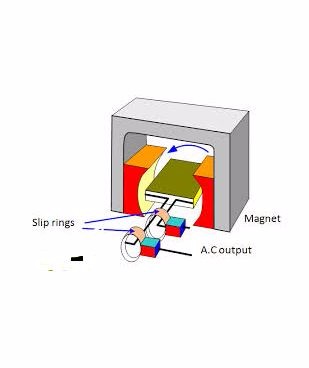When the coil is vertical, no cutting of the magnetic lines of force takes place although the number of lines linking the coil is maximum. The rate of change magnetic flux is zero and as a result, no a.m. is induced in the coil.
When the armature is parallel to the magnetic field, the rate of change of magnetic flux is maximum and the motion of the coil is perpendicular to the magnetic field, hence an a.m. is induced along the sides of the coil.
After a 180° turn, starting from the vertical position, the sides of the loop interchange and the current in the loop is reversed. This means that the a.m. is positive for one half of the cycle and negative for the half. The maximum induced a.m. is at 90° rotation from the vertical position and the minimum is at 270° rotation. If there is an external circuit, the current through it would also have a maximum value at 90° and minimum at 270°.
This kind of current is called an alternating current and the corresponding a.m. is the alternating e.m.f.The number of cycles produced per second is called the frequency of the arc. The arc obtained is led to an external circuit through the slip rings and the carbon brushes.
D.C generator
It is made by replacing the slip rings in the arc generator with a commentator. Each half of the commentator ring is called a commentator segment and is insulated from the other half. Each end of the rotating loop of the wires connected to a commentator segment. Two carbon brushes connected to the outside circuit rest against the rotating commentator.
In the deck generator, the commentator rotates with the loop of wire, just as the slip rings do with the rotor of an arc generator.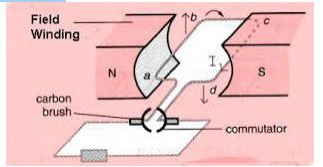When the loop is rotated in the magnetic field, the induced e.m.f is still in alternating form. However after rotation of 180° instead of the current reversing, the connections to the external circuit are reversed so that the current direction in the external circuit remains the same.
The output of a d.c generator is shown below.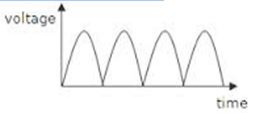Note:The lower half of the cycle is not cut off but is reversed.
Simple Step-up and Step-down Transformer
Construct a simple step-up and step-down transformer
A transformer is the device that uses mutual induction between two coils to convert an a.c across one coil to a larger or smaller a.c across the other coil.
A transformer is made up of two coils, each with a different number of loops linked by an iron core so that the magnetic flux from one passes through the other. When the flux generated by one coil changes the flux passing through the other will change, inducing a voltage in the second coil.
The coil that provides the flux that is the coil connected to the a.c power source is known as the primary coil while the coil in which the voltage is induced is known as the secondary coil.
When the number of turns in the primary coil (N) is lower than the number in the secondary coil (N),the secondary voltage will be lower than the secondary voltage. This is called the step-down transformer. The opposite of this is called the step-up transformer.
Activity 1
Construct a simple step-up and step-down transformer.

Tags

1.